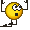# Force of spring equation problems

Elementard
Homework Statement:
A light spring having a force constant of 145 N/m is used to pull a 6.00 kg sled on a horizontal frictionless ice rink. The sled has an acceleration of 1.80 m/s2.

By how much does the spring stretch if it pulls on the sled horizontally?
Relevant Equations:
Fs= -kx
Fp= ma
m=6.00Kg
K=145N/m
a=1.80m/s

Fp=Fs
ma=-kx
(6)(1.8)=-(145)x
x=-0.0745m

I’m just wondering why I see some people make Fs=kx instead of -kx? isn’t the force of a spring a vector?

Homework Helper
Gold Member
It is a vector. Say the sled is being pulled by the spring to the right. The spring stretches to the left which means that the displacement of the end of the spring is negative. One can formally write it as a one-dimensional vector in the negative direction using unit vector notation ##\mathbf{x}=-|\mathbf{x}|~\mathbf{\hat x}##. Then one can write a vector equation for the force, $$\mathbf{F}=-k\mathbf{x}=-k(-|\mathbf{x}|~\mathbf{\hat x})=+k|\mathbf{x}|~\mathbf{\hat x}.$$ The negative sign in the one-dimensional vector equation, ##F=-kx## indicates the direction of ##F## relative to ##x##. In this example with the spring to the right of the sled, if vector ##x## is to the left (spring extension) , vector ##F## is to the right; if vector ##x## is to the right (spring compression), vector ##F## is to the left.

Last edited:
•Elementard, topsquark and TSny
Elementard
Thank you so much! This actually makes sense.👍

•kuruman
Homework Helper
Gold Member
I just noticed that your answer is a negative number. You have solved for the displacement of the end of the spring which is a vector. This is incorrect. The problem is asking you by how much the spring stretches. This is a distance, i.e. the magnitude of the displacement, and a positive number.

•Elementard and topsquark
•